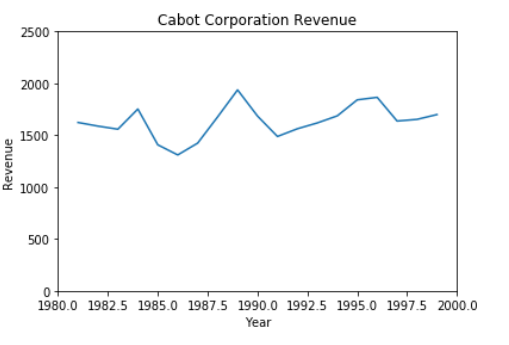# Exponential Smoothing

Exponential Smoothing is another technique to smooth the time series data to get overall long-term impression of the movements present in the data. As its name suggests, it is exponentially weighted moving averages.

Exponential smoothing is based on three terms,

• Currently observed value at time Yi
• The previously computed exponentially smoothed value Ei and
• The weight or smoothing coefficient assigned.

for the first observation in the series.

E1 = Y1
Ei = WYi + (1 – W) Ei-1 i = 2, 3, 4 …
Ei = Exponentially smooth series at time i
W = Weight or smoothing coefficient (where 0 < W < 1)
Ei-1 = Exponentially smooth series at time i-1

This method has below two advantages over Moving Average method.

• We can use it to get the short-term (one period into the future) forecast when it is questionable whether long-term trend is present in the series or not.
• Throughout the series each smoothing calculations is dependent on all previously observed values.(1 – W),Ei-1). Moving average does not consider all observed values.

Forecasting using Exponential Smoothing can be done as follow:

Ŷi+1 = Ei
Ŷi+2 = E2000 = WYi-1 + (1 – W) Ŷi-1

For example, we have revenue till 1999 and we want to make forecast for the year 2000 and 2001.

Ŷ2000 = E1999
Ŷ2001 = WY2000 + (1 – W) Ŷ2000

The choice of weight (W) is subjective. If you want to smooth the series by eliminating cyclical and irregular patterns, choose weight (W) close to 0. If you want to make the forecast, choose the weight (W) close to 1.

Let’s implement the Exponential Smoothing method using Cabot Corporation revenues using Python.

import numpy as np
import pandas as pd import matplotlib.pyplot as plt
import seaborn as sns

# Load the input files

cabot = pd.read_excel("./Data/CABOT.xls", sheet_name = "Data")[["Year", "Revenue"]]

Year Revenue
0 1981 1622.8
1 1982 1587.7
2 1983 1558.0
3 1984 1752.5
4 1985 1407.5

# Plot the Cabot Corp. Revenue

sns.lineplot(x = ("Year", y = "Revenue"), data = cabot)
plt.ylim(0,2500)
plt.xlim(1980,2000) plt.title("Cabot Corporation Revenue")Techinfoplace is a leading web solutions company that can provide you a complete range of products & solutions which help in improving your business by attracting more customers to raise your profits.

## Attended Events• Booth No.: E - 510
• EXHIBITION : 12 - 14 OCT 2017
• The Bombay Exhibition Centre at Goregaon East